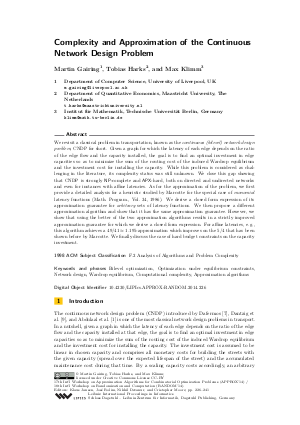Document# Complexity and Approximation of the Continuous Network Design Problem

### Authors Martin Gairing, Tobias Harks, Max Klimm## File

LIPIcs.APPROX-RANDOM.2014.226.pdf
• Filesize: 0.55 MB
• 16 pages

## Cite As

Martin Gairing, Tobias Harks, and Max Klimm. Complexity and Approximation of the Continuous Network Design Problem. In Approximation, Randomization, and Combinatorial Optimization. Algorithms and Techniques (APPROX/RANDOM 2014). Leibniz International Proceedings in Informatics (LIPIcs), Volume 28, pp. 226-241, Schloss Dagstuhl - Leibniz-Zentrum für Informatik (2014)
https://doi.org/10.4230/LIPIcs.APPROX-RANDOM.2014.226

## Abstract

We revisit a classical problem in transportation, known as the continuous (bilevel) network design problem, CNDP for short. Given a graph for which the latency of each edge depends on the ratio of the edge flow and the capacity installed, the goal is to find an optimal investment in edge capacities so as to minimize the sum of the routing cost of the induced Wardrop equilibrium and the investment cost for installing the capacity. While this problem is considered as challenging in the literature, its complexity status was still unknown. We close this gap showing that CNDP is strongly NP-complete and APX-hard, both on directed and undirected networks and even for instances with affine latencies. As for the approximation of the problem, we first provide a detailed analysis for a heuristic studied by Marcotte for the special case of monomial latency functions (Math. Program., Vol. 34, 1986). We derive a closed form expression of its approximation guarantee for arbitrary sets of latency functions. We then propose a different approximation algorithm and show that it has the same approximation guarantee. However, we show that using the better of the two approximation algorithms results in a strictly improved approximation guarantee for which we derive a closed form expression. For affine latencies, e.g., this algorithm achieves a 49/41-approximation which improves on the 5/4 that has been shown before by Marcotte. We finally discuss the case of hard budget constraints on the capacity investment.
##### Keywords
• Bilevel optimization
• Optimization under equilibrium constraints
• Network design
• Wardrop equilibrium
• Computational complexity
• Approximation algorit

## Metrics

• Access Statistics
• Total Accesses (updated on a weekly basis)
0